Next: Tracking in a solenoidal Up: Simulation by ABEL Previous: Simulation by ABEL

## Beam-beam effect

In order to investigate our method quantitatively by taking into account all beam-beam effects, which include beam disruption, the horizontal crossing angle, the vertical displacement between two beams, transverse rotations for flat beams and the energy and angular distributions of created pairs etc., we must rely on Monte-Carlo simulations by using ABEL, although the principal dynamics can be well described analytically by an approximation of the elliptic cylinder, as demonstrated in the previous section. A detailed explanation of ABEL can be found in  [6, 4]. As an example of a future linear collider we consider JLC-I, whose parameters relevant to the simulation are given as follows: the beam energy (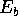) is 250GeV and the intensity (N) is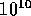/bunch; Gaussian beams with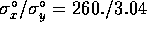nm,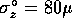m, the horizontal crossing angle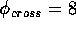mrad and the luminosity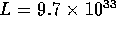cm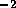sec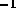with 72 bunches/pulse at the frequency of 150 Hz.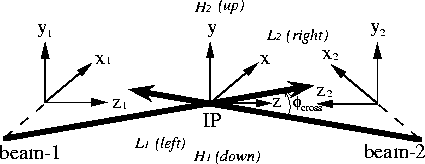Figure 2:   Coordinate system used in ABEL, where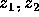and z are collinear. The trajectories of the beam-centers are indicated by the long/thick arrows.

We first define the coordinate system used in ABEL. There are three kinds of coordinate systems shown in Fig.2. Two of them,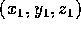and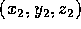, move together with beam-1 (positrons) and beam-2 (electrons), respectively. The former is a left-handed system, while the latter is a right-handed system. The third one, (x,y,z), is the rest frame at IP. All three coordinates are collinear in the z-direction. In the presence of crossing, the origins of the moving systems are shifted from the centers of the beams, except in the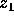- and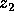- directions, as shown in Fig.2. The crossing angles of two beams are +4mrad, each in the directions of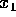and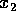, that is 8mrad (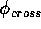) between them. Since we expect a large asymmetry in the azimuthal angular distribution of the deflected particles, especially in the vertical-horizontal asymmetry for flat beams, as explained in the previous section, we define 4 azimuthal angular regions in Fig.2 (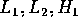and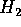) corresponding to left, right, down and up, respectively, as seen from the upstream of beam-2.

The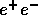pairs are generated from three processes (BH, BW and LL), where the energies of the particles are more than 5 MeV; they are tracked with all of the effects during a beam-beam crossing by ABEL. After the crossing, the azimuthal angular distribution is plotted for particles whose energies (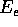) and deflection angles (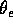) are more than 100 MeV and 20 mrad, respectively, in Fig.3 (a),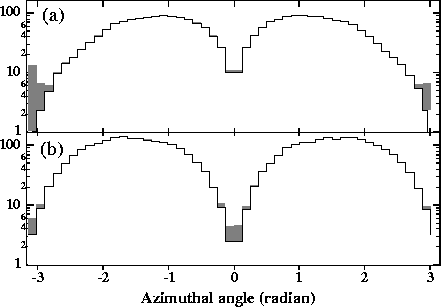Figure 3:   (a) Azimuthal angular distribution of deflected electrons and positrons(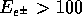MeV,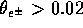) for JLC-I. The shaded area corresponds to that of those positrons which are oppositely charged particles as that of the oncoming beam. (b) The same as (a) except for a zero horizontal crossing angle, i.e.  for a head-on collision. The vertical scale is the number of particles per single-bunch crossing.

which is compared to the case of a head-on collision, i.e.  the zero horizontal crossing angles shown in Fig.3 (b). Hereafter, we always show the azimuthal angles measured downstream from beam-1, that is, those of the particles deflected by beam-2, since the distributions are symmetrical at both sides of IP for the same parameters of the two beams. As can be clearly seen in Fig.3, electrons of the pairs, which have the same charge as those of beam-2, are strongly deflected in the vertical direction, while the positrons show only a moderate suppression in the horizontal plane, as indicated by the shaded area in this figure. We can also see the effect of the 8mrad crossing with a tendency of deflecting more to the right. This shows a general feature, that is, when particles have a finite initial angle they are deflected more in this direction. The crossing angle generates a horizontal displacement (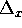) uniformly over the particles at a very early stage of their deflections. The certain displacement ofis the origin of this feature, as mentioned in the previous section.

Since the created particles have inherent scattering angles (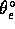) of typically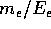, where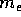is the electron mass, an effect due to these angles is also expected. It has been found to produce a deeper depletion in the horizontal direction than speculated in the previous section, where the inherent angle was neglected. F(0) (also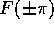) is now nearly proportional to 1/R rather than to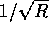in Eq.(13), as can be seen in Fig.4.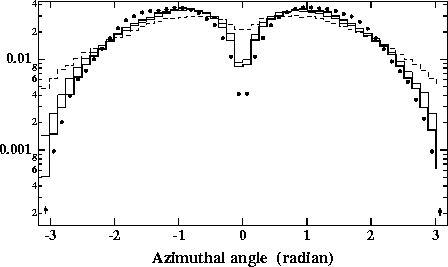Figure 4:   Azimuthal angular distributions of deflected electrons (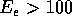MeV,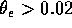), whose charges are the same as that of beam-2 for JLC-I with nominal parameters (solid circles) together with three cases (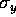=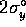(solid line),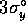(dotted one) and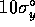(dashed one)) in which the other beam parameters are the same as those of the nominal beam. The distributions were normalized by the total number of electrons in them.

In this figure the azimuthal angular distribution for the nominal JLC-I parameters is compared with three cases (=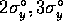and), where the other parameters are the same as the nominal ones and only those electrons ofMeV andare plotted in order to see the depletion more clearly as a function of.Next: Tracking in a solenoidal Up: Simulation by ABEL Previous: Simulation by ABEL

Toshiaki Tauchi
Sat Dec 21 00:34:16 JST 1996